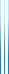# PublicationsManuscripts

## Expositions

Yuval Filmus, Intersecting families are approximately contained in juntas
Yuval Filmus, Berge's theorem on ideals of sets
Yuval Filmus, Harper's isoperimetric inequality
Yuval Filmus, Forcing with random variables and proof complexity
Yuval Filmus, Reckhow's theorem
Yuval Filmus, Smolensky's polynomial method

## TSS Lectures

Yuval Filmus, Submodular maximization
Yuval Filmus, Permanent is hard to compute even on a good day
Yuval Filmus, Matrix multiplication
Yuval Filmus, Hardness of approximating set cover
Yuval Filmus, Two proofs of the central limit theorem
Yuval Filmus, Modern integer factorization methods
Yuval Filmus, Seven trees in one

## Short Notes

Yuval Filmus and Nathan Lindzey, Murali's basis for the slice
Yuval Filmus, One-step modified log Sobolev on the complete complex
Yuval Filmus, Information complexity of AND
Yuval Filmus, NPN equivalence classes of Boolean functions
Yuval Filmus, Antichains on the Boolean lattice of dimension 6
Yuval Filmus, Khintchine–Kahane using Fourier analysis
Yuval Filmus, Triangle-intersecting families of graphs on eight vertices
Yuval Filmus, Regular languages closed under Kleene plus
Yuval Filmus, Proof of the $p$-biased version of the Erdős–Ko–Rado theorem using Katona's method
Yuval Filmus, Thirteenth proof of a result about tiling a rectangle
Yuval Filmus, Self-avoiding walks on the integers which move at most two integers at a time
Yuval Filmus, Range of symmetric matrices mod 2
Yuval Filmus, On the number of NOT gates needed to invert n inputs
Yuval Filmus, On the sequence $n$ mod $x$
Yuval Filmus, Permutations avoiding patterns of length 3
Yuval Filmus, Equivalent definitions of the Sprague–Grundy function
Yuval Filmus, Examples of the GCD proof system
Yuval Filmus, Until cannot be expressed using Next, Always, Eventually
Yuval Filmus, Orthogonal matrices with optimal $L_2$ norm
Yuval Filmus, Largest adjacent segments on the unit circle
Yuval Filmus, A positive proof of Dehn's theorem
Yuval Filmus, A combinatorial interpretation for the product of two geometric series in independent variables
Yuval Filmus, Riddle concerning $\pm1$ vectors

## Classics

Alex Wilkie, Wilkie's proof of the switching lemma
McKay's easy proof of Cauchy's theorem in group theory
Adolf Hurwitz, Hurwitz's proof that four-square-like identities only occur in dimensions 1,2,4,8
Adolf Hurwitz, Hurwitz's proof of the transcendence of $e$
Paul Gordan, Gordan's proof of the transcendence of $e$ and $\pi$
David Hilbert, Hilbert's proof of the transcendence of $e$ and $\pi$
Joseph-Louis Lagrange, Lagrange's proof of Wilson's theorem
Joseph-Louis Lagrange, Lagrange's proof of the four square theorem